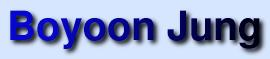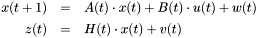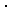Boyoon Jung's Library Manual

# bj::KalmanFilter Class Reference [Kalman Filter]

A Kalman Filter class for linear systems. More...

#include <kalman.h>

List of all members.

## Public Member Functions

KalmanFilter (int stateDim, int inputDim, int *measurementDim, int size)
A constructor.
KalmanFilter (int stateDim, int inputDim, int measurementDim)
A constructor.
KalmanFilter (void)
A constructor.
~KalmanFilter (void)
A destructor.
void initialize (gsl_vector *x, gsl_matrix *P, gsl_matrix *A=0, gsl_matrix *B=0, gsl_matrix *Q=0, gsl_matrix **H=0, gsl_matrix **R=0, int size=0)
Set parameters.
void initialize (gsl_vector *x, gsl_matrix *P, gsl_matrix *A, gsl_matrix *B, gsl_matrix *Q, gsl_matrix *H, gsl_matrix *R)
Set parameters.
void timeUpdate (gsl_vector *u, gsl_matrix *A=0, gsl_matrix *B=0, gsl_matrix *Q=0)
Evaluate the time update equation. [prediction step].
void measurementUpdate (gsl_vector *z, gsl_matrix *H=0, gsl_matrix *R=0, int index=0)
Evaluate the measurement update equation. [correction step].
void measurementUpdate (gsl_vector *z, int index)
Evaluate the measurement update equation. [correction step].
void update (gsl_vector *u, gsl_vector *z, gsl_matrix *A=0, gsl_matrix *B=0, gsl_matrix *Q=0, gsl_matrix *H=0, gsl_matrix *R=0, int index=0)
Evaluate the time & the measurement update equations.
void udpate (gsl_vector *u, gsl_vector *z, int index)
Evaluate the time & the measurement update equations.
gsl_vector * state (void)
Retrieve the current state estimate.
gsl_matrix * covariance (void)
Retrieve the current error covariance.

## Protected Member Functions

void gsl_matrix_inverse (gsl_matrix *org, gsl_matrix *inv, int index)
Compute the inverse matrix of a given square matrix.

## Protected Attributes

int msize
The number of measurements.
gsl_matrix * A
Process model.
gsl_matrix * B
Process model.
gsl_matrix * Q
Process noise model.
gsl_matrix ** H
Measurement model.
gsl_matrix ** R
Measurement noise model.
gsl_vector * x
State estimate.
gsl_matrix * P
Error covariance.
gsl_matrix ** K
Kalman gain.
gsl_permutation ** pi
int si
gsl_vector * t0_n1
gsl_matrix * t0_nn
gsl_matrix * t1_nn
gsl_vector ** t0_m1
gsl_matrix ** t0_mm
gsl_matrix ** t1_mm
gsl_matrix ** t0_nm

## Detailed Description

A Kalman Filter class for linear systems.

• Dynamic equations:• Noise distribution:## Constructor & Destructor Documentation

 bj::KalmanFilter::KalmanFilter ( int stateDim, int inputDim, int * measurementDim, int size )

A constructor.

This constructor is for when there are multiple measurements.

Parameters:
 stateDim The dimension of a state vector. inputDim The dimension of a control input vector. measurementDim The dimensions of measurement vectors. size The number of measurements.

 bj::KalmanFilter::KalmanFilter ( int stateDim, int inputDim, int measurementDim )

A constructor.

This constructor is for when there is a single measurement.

Parameters:
 stateDim The dimension of a state vector. inputDim The dimension of a control input vector. measurementDim The dimension of a measurement vector.

 bj::KalmanFilter::KalmanFilter ( void )
 A constructor.

 bj::KalmanFilter::~KalmanFilter ( void )
 A destructor.

## Member Function Documentation

 gsl_matrix* bj::KalmanFilter::covariance ( void )  [inline]
 Retrieve the current error covariance.

 void bj::KalmanFilter::gsl_matrix_inverse ( gsl_matrix * org, gsl_matrix * inv, int index )  [inline, protected]
 Compute the inverse matrix of a given square matrix. The original matrix will be destroyed !!!

 void bj::KalmanFilter::initialize ( gsl_vector * x, gsl_matrix * P, gsl_matrix * A, gsl_matrix * B, gsl_matrix * Q, gsl_matrix * H, gsl_matrix * R )  [inline]

Set parameters.

This is for when there is a single measurement.

Parameters:
 x Initial state estimate. P Initial error covariance. A Transition matrix. B Input matrix. Q Covariance of process noise. H Measurement matrix. R Covariance of measurement noise.

 void bj::KalmanFilter::initialize ( gsl_vector * x, gsl_matrix * P, gsl_matrix * A = 0, gsl_matrix * B = 0, gsl_matrix * Q = 0, gsl_matrix ** H = 0, gsl_matrix ** R = 0, int size = 0 )

Set parameters.

This is for when there are multiple measurements.

Parameters:
 x Initial state estimate. P Initial error covariance. A Transition matrix. B Input matrix. Q Covariance of process noise. H Measurement matrix. R Covariance of measurement noise. size The number of measurements.

 void bj::KalmanFilter::measurementUpdate ( gsl_vector * z, int index )  [inline]

Evaluate the measurement update equation. [correction step].

Parameters:
 z Measurement. index The index of a measurement when there are multiple measurements available.

 void bj::KalmanFilter::measurementUpdate ( gsl_vector * z, gsl_matrix * H = 0, gsl_matrix * R = 0, int index = 0 )

Evaluate the measurement update equation. [correction step].

Parameters:
 z Measurement. H Measurement matrix. R Covariance of measurement noise. index The index of a measurement when there are multiple measurements available.

 gsl_vector* bj::KalmanFilter::state ( void )  [inline]
 Retrieve the current state estimate.

 void bj::KalmanFilter::timeUpdate ( gsl_vector * u, gsl_matrix * A = 0, gsl_matrix * B = 0, gsl_matrix * Q = 0 )

Evaluate the time update equation. [prediction step].

Parameters:
 u Control input. A Transition matrix. B Input matrix. Q Covariance of process noise.

 void bj::KalmanFilter::udpate ( gsl_vector * u, gsl_vector * z, int index )  [inline]

Evaluate the time & the measurement update equations.

This function performs a full update cycle. It evaluate the time update equation first, and the measurement update is following.

Parameters:
 u Control input. z Measurement. index The index of a measurement.

 void bj::KalmanFilter::update ( gsl_vector * u, gsl_vector * z, gsl_matrix * A = 0, gsl_matrix * B = 0, gsl_matrix * Q = 0, gsl_matrix * H = 0, gsl_matrix * R = 0, int index = 0 )  [inline]

Evaluate the time & the measurement update equations.

This function performs a full update cycle. It evaluate the time update equation first, and the measurement update is following.

Parameters:
 u Control input. z Measurement. A Transition matrix. B Input matrix. Q Covariance of process noise. H Measurement matrix. R Covariance of measurement noise. index The index of a measurement.

## Member Data Documentation

 gsl_matrix* bj::KalmanFilter::A [protected]
 Process model.

 gsl_matrix* bj::KalmanFilter::B [protected]
 Process model.

 gsl_matrix** bj::KalmanFilter::H [protected]
 Measurement model.

 gsl_matrix** bj::KalmanFilter::K [protected]
 Kalman gain.

 int bj::KalmanFilter::msize [protected]
 The number of measurements.

 gsl_matrix* bj::KalmanFilter::P [protected]
 Error covariance.

 gsl_permutation** bj::KalmanFilter::pi [protected]

 gsl_matrix* bj::KalmanFilter::Q [protected]
 Process noise model.

 gsl_matrix** bj::KalmanFilter::R [protected]
 Measurement noise model.

 int bj::KalmanFilter::si [protected]

 gsl_vector** bj::KalmanFilter::t0_m1 [protected]

 gsl_matrix** bj::KalmanFilter::t0_mm [protected]

 gsl_vector* bj::KalmanFilter::t0_n1 [protected]

 gsl_matrix** bj::KalmanFilter::t0_nm [protected]

 gsl_matrix* bj::KalmanFilter::t0_nn [protected]

 gsl_matrix ** bj::KalmanFilter::t1_mm [protected]

 gsl_matrix * bj::KalmanFilter::t1_nn [protected]

 gsl_vector* bj::KalmanFilter::x [protected]
 State estimate.

The documentation for this class was generated from the following file:
ResearchProjectsPublications

ToolsBjLib ManualPlayer/StagePyPlayerMicroStrain IMUGPS/DGPSParticle Filter

PersonalWelcomeCurriculum VitaeResumeContact

Outside LinksUSCUSC CS DeptCRESRobotics LabRESL